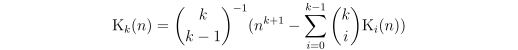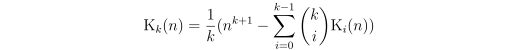# Closed-Form Expessions for Summations

Last modification on

## Abstract

Some mathematical concepts are most intuitively described using summations. To efficiently calculate and work with the results of such summations, it may be necessary to represent them as a closed-form expression. The following describes a method for finding closed-form expressions of summations: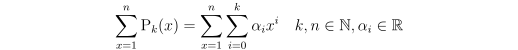## Method

The first thing to note is that a summation over a polynomial may be decomposed into a sum over the summations of the individual polynomial components: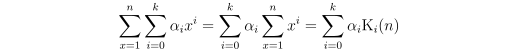To discover a closed-form expression for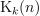we will represent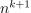as a summation, which must consist of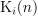with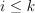. By iteratively calculating and subtractingfor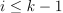, we are left with a closed-form expression for.

## Example

Let's have a look at how a closed-form expression for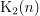may be derived without prior knowledge offor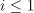.

We begin by representingas a summation. We can represent any power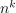by "integrating" over the differences in consecutive values: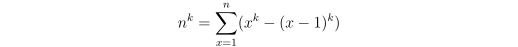For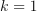: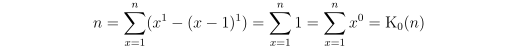For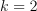: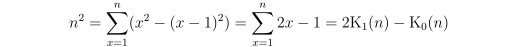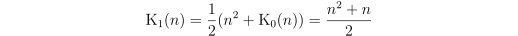For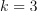: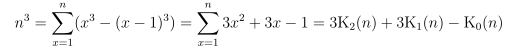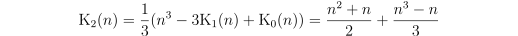## Generalization

We can use binomial coefficients to represent the difference in powers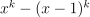in terms of a summation over the polynomial components: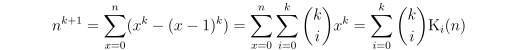Solving for: